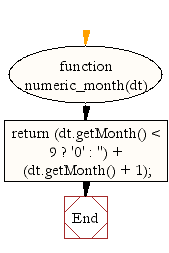# JavaScript: Get a numeric representation of a month

## JavaScript Datetime: Exercise-26 with Solution

Write a JavaScript function to get a numeric representation of a month, with leading zeros (01 through 12).

Test Data:
dt = new Date(2015, 10, 1);
console.log(numeric_month(dt));
"11"

Sample Solution:-

HTML Code:

``````<!DOCTYPE html>
<html>
<meta charset="utf-8">
<title>JavaScript function to get a numeric representation of a month, with leading zeros (01 through 12).</title>
<body>

</body>
</html>
```
```

JavaScript Code:

``````function numeric_month(dt)
{
return (dt.getMonth() < 9 ? '0' : '') + (dt.getMonth() + 1);
}
dt = new Date();
console.log(numeric_month(dt));

dt = new Date(2015, 10, 1);
console.log(numeric_month(dt));
```
```

Sample Output:

```06
11
```

Flowchart:Live Demo:

See the Pen JavaScript - Get a numeric representation of a month-date-ex-26 by w3resource (@w3resource) on CodePen.

Improve this sample solution and post your code through Disqus

What is the difficulty level of this exercise?

Test your Programming skills with w3resource's quiz.

﻿

## JavaScript: Tips of the Day

Add padding to the beginning of a string

```const name = 'Minta Lofton';